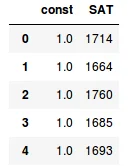31 May 2022

Posted on:

25 Apr 2020

0

Instructor
Posted on:

25 Apr 2020

2
Hi Anuridh, Thanks for reaching out. OLS requires the input to have a column of ones. We need to add this column manually.
``x = sm.add_constant(x1)` `
adds a column of ones to the `x1` array (`data['SAT']`). Here is the head of `x`:As you can see, a column of ones is added to `SAT`. This column of ones corresponds to x_0 in the simple linear regression equation: y_hat = b_0 * x_0 + b_1 * x_1. As explained in the lecture video, x_0 is always one and thus the regression equation becomes: y_hat = b_0 * 1 + b_1 * x_1. The `x` array holds `1` and `x_1`. Best,
The 365 Team
Posted on:

31 May 2022

0

thanks for the answer data365 team, currently learn your course on udemy. and im dying to know what is add_contsant do lol